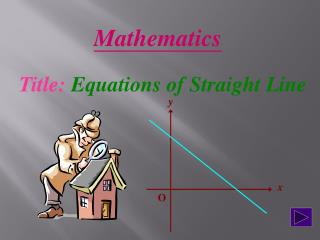DownloadDownload PresentationMathematics

# Mathematics

Télécharger la présentation## Mathematics

- - - - - - - - - - - - - - - - - - - - - - - - - - - E N D - - - - - - - - - - - - - - - - - - - - - - - - - - -
##### Presentation Transcript

1. y x O Mathematics Title: Equations of Straight Line

2. Slope of AB = Slope of PA = Two-Point Form y Given a straight line which passes through the points A and B, then x x x If P(x, y) is any point on the line AB, then x O

3. = Since PA and AB are parts of the same straight line, then Slope of PA = Slope of AB i.e. This equation is known as the Two-Point Form of the straight line. (Go to Example 1)

4. y Slope of PA = x O Point-Slope Form Given a straight line which passes through the point A and has m as its slope. x Slope = m If P(x, y) is any point on the line , then x

5. = m Since slope of PA equals to the slope of the straight line, then i.e. This equation is known as the Point-Slope Form of the straight line. (Go to Example 2)

6. y x O Slope of PA = Slope-Intercept Form Given a straight line which cuts the y-axis at A and with slope m . (Note: c is called the y-intercept of the straight line.) x P(x, y) A(0, c) x c If P is any point on the line, then Slope = m

7. Since slope of PA is equal to the slope of the line, then by Point-Slope Form (y - c) = m(x – 0) y = mx + c i.e. This equation is known as the Slope-Intercept Form of the straight line. (Go to Example 3)

8. y Slope of AB = = x O Slope of PA = Intercept Form Given a straight line which cuts the x-axis at A and y-axis at B. (Note: a is called the x-intercept of the straight line.) B(0, b) x P(x, y) b x A(a, 0) x a If P is any point on the line, then

9. = Since the slope of PA equals to the slope of AB, then bx + ay = ab Dividing both sides by ab, we have This equation is known as the Intercept Form of the straight line. (Go to Example 4)

10. Standard Form It should be noted that all the different standard forms of the equation of a straight line can be reduced to the form Ax + By + C = 0 where A, B and C are constants with A and B not both zero. This equation is known as the Standard Form of a straight line.

11. y x O Mathematics Linear Equations

12. y x O Example 1 (Two-Point Form) Find the equation of the straight line. B(5, 6) x The required equation: x x A(1, 3) 4(y – 3) = 3(x – 1) i.e. 3x – 4y + 9 = 0 (Note: The answer is in General Form)

13. y x O Example 2 (Point-Slope Form) Find the equation of the straight line. x Slope = 2 P(x, y) The required equation: y – 3 = 2(x – 1) x A(1, 3) i.e. 2x – y + 1 = 0 (Note: The answer is in General Form)

14. y x O Example 3 (Slope-Intercept Form) Find the equation of the straight line. P(x, y) x The required equation: A(0, 4) x y = 2x + 4 i.e. 2x – y + 4 = 0 Slope = 2 (Note: The answer is in General Form)

15. y x O Example 4 (Standard Form) Find the equation of the straight line. The required equation: B(0, 5) x P(x, y) 5x + 7y = 35 x A(7, 0) i.e. 5x + 7y – 35 = 0 x (Note: The answer is in Standard Form)

16. THE END